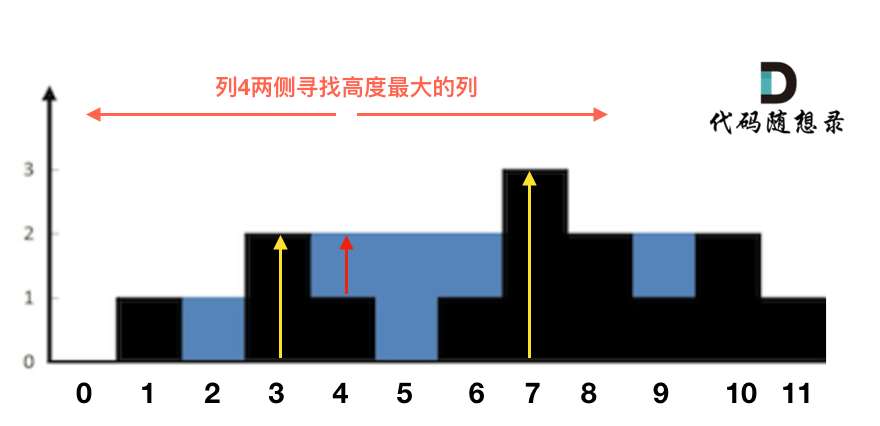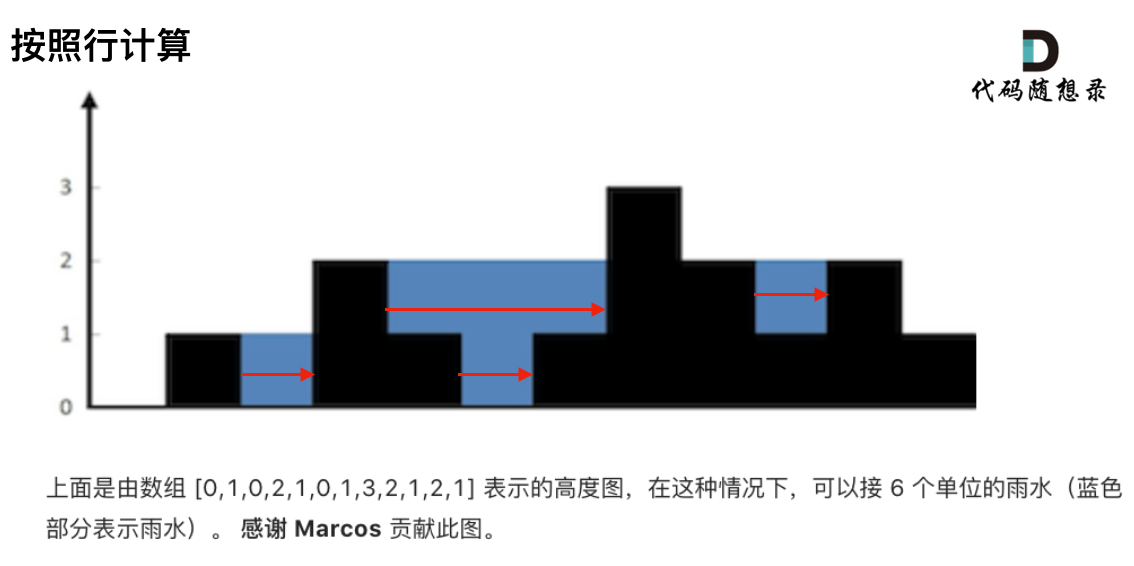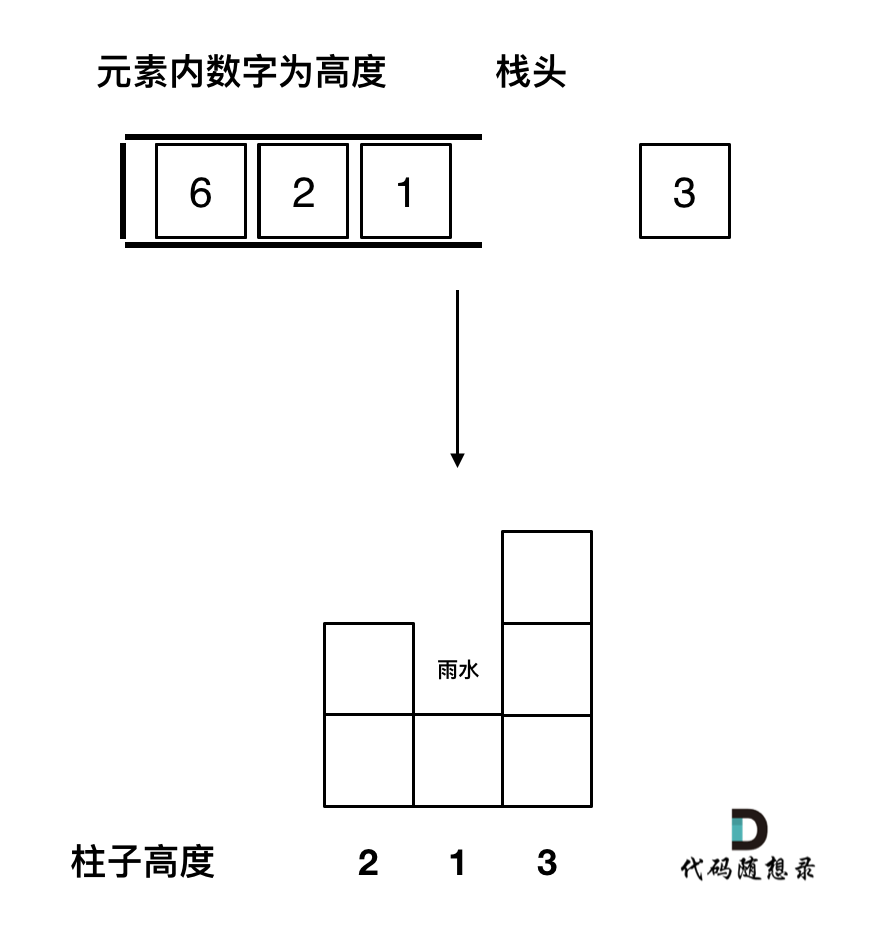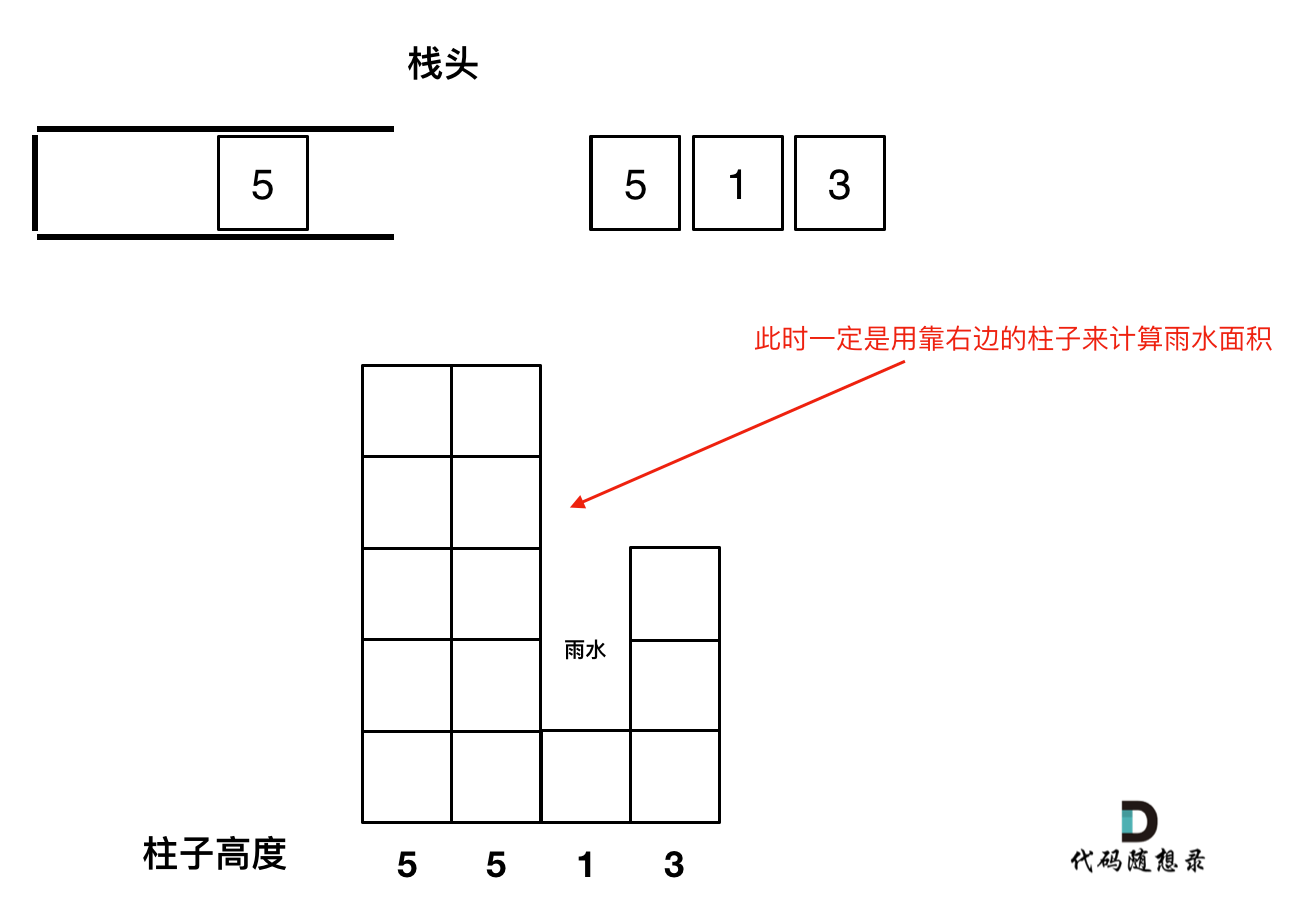# # 42. 接雨水• 输入：height = [0,1,0,2,1,0,1,3,2,1,2,1]
• 输出：6
• 解释：上面是由数组 [0,1,0,2,1,0,1,3,2,1,2,1] 表示的高度图，在这种情况下，可以接 6 个单位的雨水（蓝色部分表示雨水）。

• 输入：height = [4,2,0,3,2,5]
• 输出：9

# # 思路

• 双指针法
• 动态规划
• 单调栈

## # 双指针解法``````for (int i = 0; i < height.size(); i++) {
// 第一个柱子和最后一个柱子不接雨水
if (i == 0 || i == height.size() - 1) continue;
}
``````
1
2
3
4

``````int rHeight = height[i]; // 记录右边柱子的最高高度
int lHeight = height[i]; // 记录左边柱子的最高高度
for (int r = i + 1; r < height.size(); r++) {
if (height[r] > rHeight) rHeight = height[r];
}
for (int l = i - 1; l >= 0; l--) {
if (height[l] > lHeight) lHeight = height[l];
}
``````
1
2
3
4
5
6
7
8

``````int h = min(lHeight, rHeight) - height[i];
if (h > 0) sum += h; // 注意只有h大于零的时候，在统计到总和中
``````
1
2

``````class Solution {
public:
int trap(vector<int>& height) {
int sum = 0;
for (int i = 0; i < height.size(); i++) {
// 第一个柱子和最后一个柱子不接雨水
if (i == 0 || i == height.size() - 1) continue;

int rHeight = height[i]; // 记录右边柱子的最高高度
int lHeight = height[i]; // 记录左边柱子的最高高度
for (int r = i + 1; r < height.size(); r++) {
if (height[r] > rHeight) rHeight = height[r];
}
for (int l = i - 1; l >= 0; l--) {
if (height[l] > lHeight) lHeight = height[l];
}
int h = min(lHeight, rHeight) - height[i];
if (h > 0) sum += h;
}
return sum;
}
};
``````
1
2
3
4
5
6
7
8
9
10
11
12
13
14
15
16
17
18
19
20
21
22

## # 动态规划解法

``````class Solution {
public:
int trap(vector<int>& height) {
if (height.size() <= 2) return 0;
vector<int> maxLeft(height.size(), 0);
vector<int> maxRight(height.size(), 0);
int size = maxRight.size();

// 记录每个柱子左边柱子最大高度
maxLeft = height;
for (int i = 1; i < size; i++) {
maxLeft[i] = max(height[i], maxLeft[i - 1]);
}
// 记录每个柱子右边柱子最大高度
maxRight[size - 1] = height[size - 1];
for (int i = size - 2; i >= 0; i--) {
maxRight[i] = max(height[i], maxRight[i + 1]);
}
// 求和
int sum = 0;
for (int i = 0; i < size; i++) {
int count = min(maxLeft[i], maxRight[i]) - height[i];
if (count > 0) sum += count;
}
return sum;
}
};
``````
1
2
3
4
5
6
7
8
9
10
11
12
13
14
15
16
17
18
19
20
21
22
23
24
25
26
27

## # 单调栈解法

### # 准备工作

1. 首先单调栈是按照行方向来计算雨水，如图：1. 使用单调栈内元素的顺序1. 遇到相同高度的柱子怎么办。1. 栈里要保存什么数值

``````stack<int> st; // 存着下标，计算的时候用下标对应的柱子高度
``````
1

### # 单调栈处理逻辑

``````if (height[i] < height[st.top()])  st.push(i);
``````
1

``````if (height[i] == height[st.top()]) { // 例如 5 5 1 7 这种情况
st.pop();
st.push(i);
}
``````
1
2
3
4``````while (!st.empty() && height[i] > height[st.top()]) { // 注意这里是while，持续跟新栈顶元素
int mid = st.top();
st.pop();
if (!st.empty()) {
int h = min(height[st.top()], height[i]) - height[mid];
int w = i - st.top() - 1; // 注意减一，只求中间宽度
sum += h * w;
}
}
``````
1
2
3
4
5
6
7
8
9

``````class Solution {
public:
int trap(vector<int>& height) {
if (height.size() <= 2) return 0; // 可以不加
stack<int> st; // 存着下标，计算的时候用下标对应的柱子高度
st.push(0);
int sum = 0;
for (int i = 1; i < height.size(); i++) {
if (height[i] < height[st.top()]) {     // 情况一
st.push(i);
} if (height[i] == height[st.top()]) {  // 情况二
st.pop(); // 其实这一句可以不加，效果是一样的，但处理相同的情况的思路却变了。
st.push(i);
} else {                                // 情况三
while (!st.empty() && height[i] > height[st.top()]) { // 注意这里是while
int mid = st.top();
st.pop();
if (!st.empty()) {
int h = min(height[st.top()], height[i]) - height[mid];
int w = i - st.top() - 1; // 注意减一，只求中间宽度
sum += h * w;
}
}
st.push(i);
}
}
return sum;
}
};
``````
1
2
3
4
5
6
7
8
9
10
11
12
13
14
15
16
17
18
19
20
21
22
23
24
25
26
27
28
29

``````class Solution {
public:
int trap(vector<int>& height) {
stack<int> st;
st.push(0);
int sum = 0;
for (int i = 1; i < height.size(); i++) {
while (!st.empty() && height[i] > height[st.top()]) {
int mid = st.top();
st.pop();
if (!st.empty()) {
int h = min(height[st.top()], height[i]) - height[mid];
int w = i - st.top() - 1;
sum += h * w;
}
}
st.push(i);
}
return sum;
}
};
``````
1
2
3
4
5
6
7
8
9
10
11
12
13
14
15
16
17
18
19
20
21

## # 其他语言版本

Java:

``````class Solution {
public int trap(int[] height) {
int sum = 0;
for (int i = 0; i < height.length; i++) {
// 第一个柱子和最后一个柱子不接雨水
if (i==0 || i== height.length - 1) continue;

int rHeight = height[i]; // 记录右边柱子的最高高度
int lHeight = height[i]; // 记录左边柱子的最高高度
for (int r = i+1; r < height.length; r++) {
if (height[r] > rHeight) rHeight = height[r];
}
for (int l = i-1; l >= 0; l--) {
if(height[l] > lHeight) lHeight = height[l];
}
int h = Math.min(lHeight, rHeight) - height[i];
if (h > 0) sum += h;
}
return sum;

}
}
``````
1
2
3
4
5
6
7
8
9
10
11
12
13
14
15
16
17
18
19
20
21
22

``````class Solution {
public int trap(int[] height) {
int length = height.length;
if (length <= 2) return 0;
int[] maxLeft = new int[length];
int[] maxRight = new int[length];

// 记录每个柱子左边柱子最大高度
maxLeft = height;
for (int i = 1; i< length; i++) maxLeft[i] = Math.max(height[i], maxLeft[i-1]);

// 记录每个柱子右边柱子最大高度
maxRight[length - 1] = height[length - 1];
for(int i = length - 2; i >= 0; i--) maxRight[i] = Math.max(height[i], maxRight[i+1]);

// 求和
int sum = 0;
for (int i = 0; i < length; i++) {
int count = Math.min(maxLeft[i], maxRight[i]) - height[i];
if (count > 0) sum += count;
}
return sum;
}
}
``````
1
2
3
4
5
6
7
8
9
10
11
12
13
14
15
16
17
18
19
20
21
22
23
24

Python:

``````class Solution:
def trap(self, height: List[int]) -> int:
res = 0
for i in range(len(height)):
if i == 0 or i == len(height)-1: continue
lHight = height[i-1]
rHight = height[i+1]
for j in range(i-1):
if height[j] > lHight:
lHight = height[j]
for k in range(i+2,len(height)):
if height[k] > rHight:
rHight = height[k]
res1 = min(lHight,rHight) - height[i]
if res1 > 0:
res += res1
return res
``````
1
2
3
4
5
6
7
8
9
10
11
12
13
14
15
16
17

``````class Solution:
def trap(self, height: List[int]) -> int:
leftheight, rightheight = *len(height), *len(height)

leftheight=height
for i in range(1,len(height)):
leftheight[i]=max(leftheight[i-1],height[i])
rightheight[-1]=height[-1]
for i in range(len(height)-2,-1,-1):
rightheight[i]=max(rightheight[i+1],height[i])

result = 0
for i in range(0,len(height)):
summ = min(leftheight[i],rightheight[i])-height[i]
result += summ
return result
``````
1
2
3
4
5
6
7
8
9
10
11
12
13
14
15
16

``````class Solution:
def trap(self, height: List[int]) -> int:
st =
result = 0
for i in range(1,len(height)):
while st!=[] and height[i]>height[st[-1]]:
midh = height[st[-1]]
st.pop()
if st!=[]:
hright = height[i]
hleft = height[st[-1]]
h = min(hright,hleft)-midh
w = i-st[-1]-1
result+=h*w
st.append(i)
return result
``````
1
2
3
4
5
6
7
8
9
10
11
12
13
14
15
16

Go:

JavaScript: